# Linear Mixed-Effects Model Workflow

This example shows how to fit and analyze a linear mixed-effects model (LME).

load flu 

The flu dataset array has a Date variable, and 10 variables containing estimated influenza rates (in 9 different regions, estimated from Google® searches, plus a nationwide estimate from the CDC).

### Reorganize and plot the data.

To fit a linear-mixed effects model, your data must be in a properly formatted dataset array. To fit a linear mixed-effects model with the influenza rates as the responses, combine the nine columns corresponding to the regions into an array. The new dataset array, flu2, must have the response variable FluRate, the nominal variable Region that shows which region each estimate is from, the nationwide estimate WtdILI, and the grouping variable Date.

flu2 = stack(flu,2:10,'NewDataVarName','FluRate',... 'IndVarName','Region'); flu2.Date = nominal(flu2.Date); 

Define flu2 as a table.

flu2 = dataset2table(flu2); 

Plot flu rates versus the nationwide estimate.

plot(flu2.WtdILI,flu2.FluRate,'ro') xlabel('WtdILI') ylabel('Flu Rate')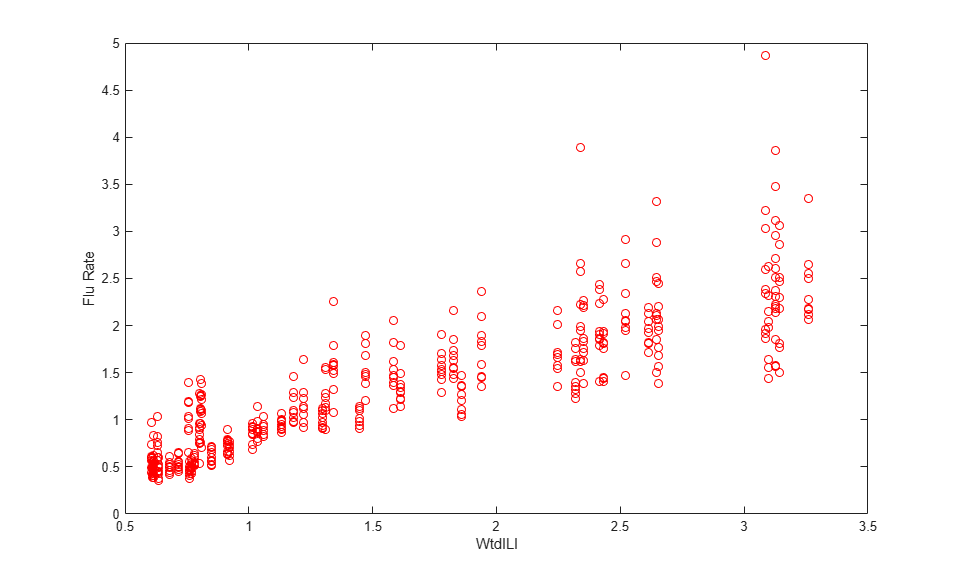You can see that the flu rates in regions have a direct relationship with the nationwide estimate.

### Fit an LME model and interpret the results.

Fit a linear mixed-effects model with the nationwide estimate as the predictor variable and a random intercept that varies by Date.

lme = fitlme(flu2,'FluRate ~ 1 + WtdILI + (1|Date)') 
lme = Linear mixed-effects model fit by ML Model information: Number of observations 468 Fixed effects coefficients 2 Random effects coefficients 52 Covariance parameters 2 Formula: FluRate ~ 1 + WtdILI + (1 | Date) Model fit statistics: AIC BIC LogLikelihood Deviance 286.24 302.83 -139.12 278.24 Fixed effects coefficients (95% CIs): Name Estimate SE tStat DF pValue {'(Intercept)'} 0.16385 0.057525 2.8484 466 0.0045885 {'WtdILI' } 0.7236 0.032219 22.459 466 3.0502e-76 Lower Upper 0.050813 0.27689 0.66028 0.78691 Random effects covariance parameters (95% CIs): Group: Date (52 Levels) Name1 Name2 Type Estimate {'(Intercept)'} {'(Intercept)'} {'std'} 0.17146 Lower Upper 0.13227 0.22226 Group: Error Name Estimate Lower Upper {'Res Std'} 0.30201 0.28217 0.32324 

The small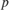-values of 0.0045885 and 3.0502e-76 indicate that both the intercept and nationwide estimate are significant. Also, the confidence limits for the standard deviation of the random-effects term,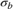, do not include 0 (0.13227, 0.22226), which indicates that the random-effects term is significant.

Plot the raw residuals versus the fitted values.

figure(); plotResiduals(lme,'fitted')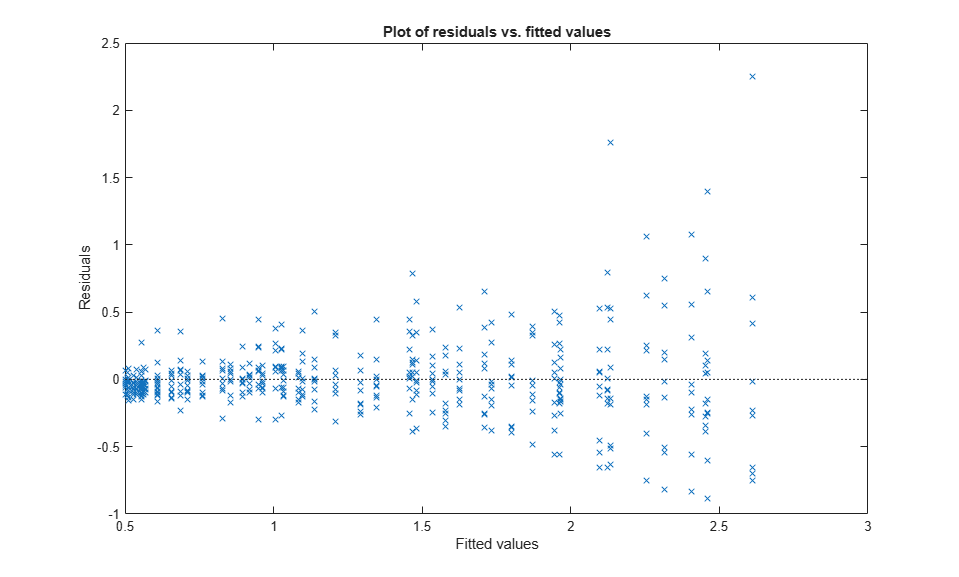The variance of residuals increases with increasing fitted response values, which is known as heteroscedasticity.

Find the two observations on the top right that appear like outliers.

find(residuals(lme) > 1.5) 
ans = 98 107 

Refit the model by removing these observations.

lme = fitlme(flu2,'FluRate ~ 1 + WtdILI + (1|Date)','Exclude',[98,107]); 

### Improve the model.

Determine if including an independent random term for the nationwide estimate grouped by Date improves the model.

altlme = fitlme(flu2,'FluRate ~ 1 + WtdILI + (1|Date) + (WtdILI-1|Date)',... 'Exclude',[98,107]) 
altlme = Linear mixed-effects model fit by ML Model information: Number of observations 466 Fixed effects coefficients 2 Random effects coefficients 104 Covariance parameters 3 Formula: FluRate ~ 1 + WtdILI + (1 | Date) + (WtdILI | Date) Model fit statistics: AIC BIC LogLikelihood Deviance 179.39 200.11 -84.694 169.39 Fixed effects coefficients (95% CIs): Name Estimate SE tStat DF pValue {'(Intercept)'} 0.17837 0.054585 3.2676 464 0.001165 {'WtdILI' } 0.70836 0.030594 23.153 464 2.123e-79 Lower Upper 0.0711 0.28563 0.64824 0.76849 Random effects covariance parameters (95% CIs): Group: Date (52 Levels) Name1 Name2 Type Estimate {'(Intercept)'} {'(Intercept)'} {'std'} 0.16631 Lower Upper 0.12977 0.21313 Group: Date (52 Levels) Name1 Name2 Type Estimate Lower {'WtdILI'} {'WtdILI'} {'std'} 4.6672e-08 NaN Upper NaN Group: Error Name Estimate Lower Upper {'Res Std'} 0.26691 0.24934 0.28572 

The estimated standard deviation of WtdILI term is nearly 0 and its confidence interval cannot be computed. This is an indication that the model is overparameterized and the (WtdILI-1|Date) term is not significant. You can formally test this using the compare method as follows: compare(lme,altlme,'CheckNesting',true).

Add a random effects-term for intercept grouped by Region to the initial model lme.

lme2 = fitlme(flu2,'FluRate ~ 1 + WtdILI + (1|Date) + (1|Region)',... 'Exclude',[98,107]); 

Compare the models lme and lme2.

compare(lme,lme2,'CheckNesting',true) 
ans = THEORETICAL LIKELIHOOD RATIO TEST Model DF AIC BIC LogLik LRStat deltaDF pValue lme 4 177.39 193.97 -84.694 lme2 5 62.265 82.986 -26.133 117.12 1 0 

The-value of 0 indicates that lme2 is a better fit than lme.

Now, check if adding a potentially correlated random-effects term for the intercept and national average improves the model lme2.

lme3 = fitlme(flu2,'FluRate ~ 1 + WtdILI + (1|Date) + (1 + WtdILI|Region)',... 'Exclude',[98,107]) 
lme3 = Linear mixed-effects model fit by ML Model information: Number of observations 466 Fixed effects coefficients 2 Random effects coefficients 70 Covariance parameters 5 Formula: FluRate ~ 1 + WtdILI + (1 | Date) + (1 + WtdILI | Region) Model fit statistics: AIC BIC LogLikelihood Deviance 13.338 42.348 0.33076 -0.66153 Fixed effects coefficients (95% CIs): Name Estimate SE tStat DF pValue {'(Intercept)'} 0.1795 0.054953 3.2665 464 0.0011697 {'WtdILI' } 0.70719 0.04252 16.632 464 4.6451e-49 Lower Upper 0.071514 0.28749 0.62363 0.79074 Random effects covariance parameters (95% CIs): Group: Date (52 Levels) Name1 Name2 Type Estimate {'(Intercept)'} {'(Intercept)'} {'std'} 0.17634 Lower Upper 0.14093 0.22064 Group: Region (9 Levels) Name1 Name2 Type Estimate {'(Intercept)'} {'(Intercept)'} {'std' } 0.0077037 {'WtdILI' } {'(Intercept)'} {'corr'} -0.059603 {'WtdILI' } {'WtdILI' } {'std' } 0.088069 Lower Upper 3.1945e-16 1.8578e+11 -0.99996 0.99995 0.051693 0.15004 Group: Error Name Estimate Lower Upper {'Res Std'} 0.20976 0.19568 0.22486 

The estimate for the standard deviation of the random-effects term for intercept grouped by Region is 0.0077037, its confidence interval is very large and includes zero. This indicates that the random-effects for intercept grouped by Region is insignificant. The correlation between the random-effects for intercept and WtdILI is -0.059604. Its confidence interval is also very large and includes zero. This is an indication that the correlation is not significant.

Refit the model by eliminating the intercept from the (1 + WtdILI | Region) random-effects term.

lme3 = fitlme(flu2,'FluRate ~ 1 + WtdILI + (1|Date) + (WtdILI - 1|Region)',... 'Exclude',[98,107]) 
lme3 = Linear mixed-effects model fit by ML Model information: Number of observations 466 Fixed effects coefficients 2 Random effects coefficients 61 Covariance parameters 3 Formula: FluRate ~ 1 + WtdILI + (1 | Date) + (WtdILI | Region) Model fit statistics: AIC BIC LogLikelihood Deviance 9.3395 30.06 0.33023 -0.66046 Fixed effects coefficients (95% CIs): Name Estimate SE tStat DF pValue {'(Intercept)'} 0.1795 0.054892 3.2702 464 0.0011549 {'WtdILI' } 0.70718 0.042486 16.645 464 4.0496e-49 Lower Upper 0.071637 0.28737 0.62369 0.79067 Random effects covariance parameters (95% CIs): Group: Date (52 Levels) Name1 Name2 Type Estimate {'(Intercept)'} {'(Intercept)'} {'std'} 0.17633 Lower Upper 0.14092 0.22062 Group: Region (9 Levels) Name1 Name2 Type Estimate Lower {'WtdILI'} {'WtdILI'} {'std'} 0.087925 0.054474 Upper 0.14192 Group: Error Name Estimate Lower Upper {'Res Std'} 0.20979 0.19585 0.22473 

All terms in the new model lme3 are significant.

Compare lme2 and lme3.

compare(lme2,lme3,'CheckNesting',true,'NSim',100) 
ans = SIMULATED LIKELIHOOD RATIO TEST: NSIM = 100, ALPHA = 0.05 Model DF AIC BIC LogLik LRStat pValue lme2 5 62.265 82.986 -26.133 lme3 5 9.3395 30.06 0.33023 52.926 0.009901 Lower Upper 0.00025064 0.053932 

The-value of 0.009901 indicates that lme3 is a better fit than lme2.

Add a quadratic fixed-effects term to the model lme3.

lme4 = fitlme(flu2,'FluRate ~ 1 + WtdILI^2 + (1|Date) + (WtdILI - 1|Region)',... 'Exclude',[98,107]) 
lme4 = Linear mixed-effects model fit by ML Model information: Number of observations 466 Fixed effects coefficients 3 Random effects coefficients 61 Covariance parameters 3 Formula: FluRate ~ 1 + WtdILI + WtdILI^2 + (1 | Date) + (WtdILI | Region) Model fit statistics: AIC BIC LogLikelihood Deviance 6.7234 31.588 2.6383 -5.2766 Fixed effects coefficients (95% CIs): Name Estimate SE tStat DF pValue {'(Intercept)'} -0.063406 0.12236 -0.51821 463 0.60456 {'WtdILI' } 1.0594 0.16554 6.3996 463 3.8232e-10 {'WtdILI^2' } -0.096919 0.0441 -2.1977 463 0.028463 Lower Upper -0.30385 0.17704 0.73406 1.3847 -0.18358 -0.010259 Random effects covariance parameters (95% CIs): Group: Date (52 Levels) Name1 Name2 Type Estimate {'(Intercept)'} {'(Intercept)'} {'std'} 0.16732 Lower Upper 0.13326 0.21009 Group: Region (9 Levels) Name1 Name2 Type Estimate Lower {'WtdILI'} {'WtdILI'} {'std'} 0.087865 0.054443 Upper 0.1418 Group: Error Name Estimate Lower Upper {'Res Std'} 0.20979 0.19585 0.22473 

The-value of 0.028463 indicates that the coefficient of the quadratic term WtdILI^2 is significant.

### Plot the fitted response versus the observed response and residuals.

F = fitted(lme4); R = response(lme4); figure(); plot(R,F,'rx') xlabel('Response') ylabel('Fitted')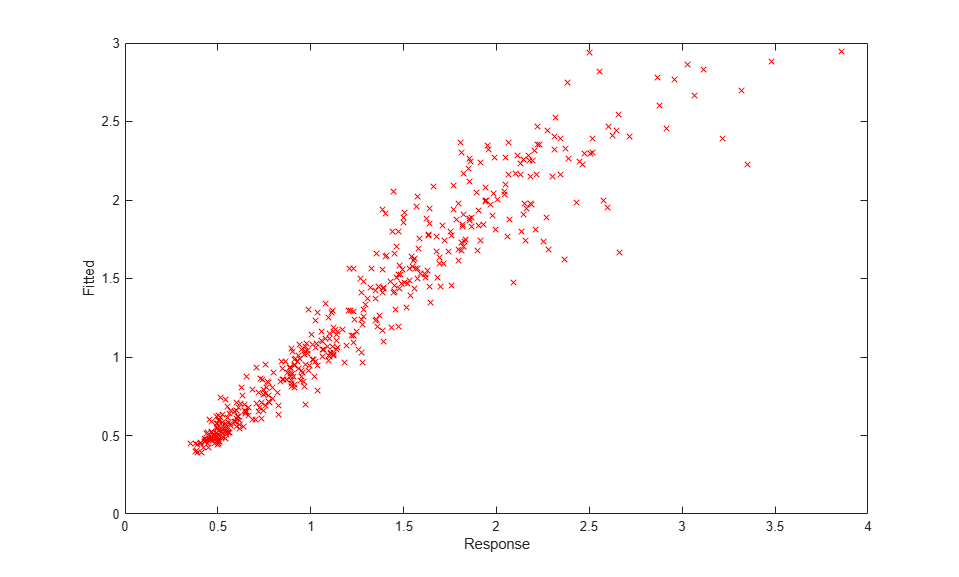The fitted versus observed response values form almost 45-degree angle indicating a good fit.

Plot the residuals versus the fitted values.

figure(); plotResiduals(lme4,'fitted')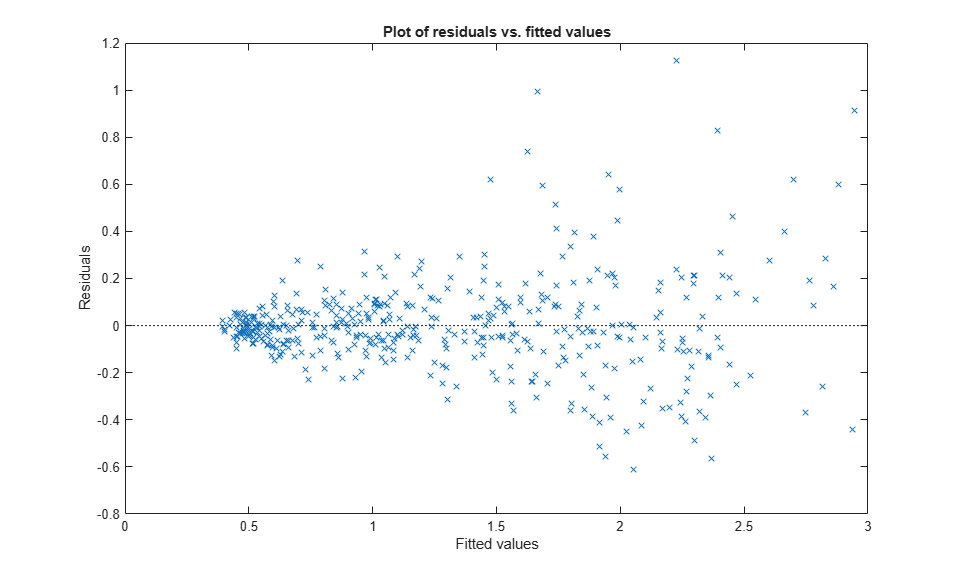Although it has improved, you can still see some heteroscedasticity in the model. This might be due to another predictor that does not exist in the data set, hence not in the model.

### Find the fitted flu rate value for region ENCentral, date 11/6/2005.

F(flu2.Region == 'ENCentral' & flu2.Date == '11/6/2005') 
ans = 1.4860 

### Randomly generate response values.

Randomly generate response values for a national estimate of 1.625, region MidAtl, and date 4/23/2006. First, define the new table. Because Date and Region are nominal in the original table, you must define them similarly in the new table.

tblnew.Date = nominal('4/23/2006'); tblnew.WtdILI = 1.625; tblnew.Region = nominal('MidAtl'); tblnew = struct2table(tblnew); 

Now, generate the response value.

random(lme4,tblnew) 
ans = 1.2679